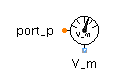Integrator - MapleSim Help

Integrator

Compute the integralDescription The Integrator component computes the element-wise integral of the input, $u$, with a multiplication gain of k. The Signal Size parameter assigns the dimension of the input and output connectors.Equations $\stackrel{.}{{y}_{j}}=k{u}_{j}$Connections

 Name Description Modelica ID $u$ Real input vector u $y$ Real output vector y $\mathrm{reset}$ Optional connector of reset signal reset $\mathrm{set}$ Optional connector of set signal setParameters

 Name Default Units Description Modelica ID Signal Size $1$ Dimension of input and output signals signalSize k $1$ Integrator gain k use reset $\mathrm{false}$ True(checked) enables reset port use_reset use set $\mathrm{false}$ True(checked) enables port that sets reset value (when use reset is selected) use_set Initial Values Initialization with initial states Type of initialization, initType ${y}_{0}$ $0$ Initial or guess value of output. y_startModelica Standard Library The component described in this topic is from the Modelica Standard Library. To view the original documentation, which includes author and copyright information, click here.